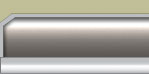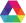#### What are CIELAB color differences?

CIE 1976 (L* a* b*) color space provides a three-dimensional representation for the perception of color stimuli. If two points in space, representing two stimuli, are coincident then the color difference between the two stimuli is zero. As the distance in space between two points increases it is reasonable to assume that the perceived color difference between the stimuli that the two points represent increases accordingly. One measure of the difference in color between two stimuli is therefore the Euclidean distance ΔE* between the two points in the three-dimensional space.
BACK

#### How good are CIELAB color differences?

Unfortunately several evaluations of CIELAB have shown that ΔE* is not a particularly good measure of the magnitude of the perceptual color difference between two stimuli. The relatively poor ability of ΔE* to predict the magnitude of perceptual color differences has led to more complicated ways of computing a color difference from the CIELAB coordinates of two samples and some of these measures have been shown to be more reliable than ΔE*.
BACK

#### How do I get descriptive color differences?

The L* C* H* representation is useful if qualitative color differences are required. Differences can be calculated thus:

ΔL* = L*btx - L*std,
ΔC*= C*btx - C*std
ΔH*= {(Δa*)2 + (Δb*)2 - (ΔC*)2}1/2

where the subscripts std and btx refer to standard and batch respectively.

If ΔL* is positive the batch is lighter than the standard, but if ΔL* is negative the batch is darker than the standard.

If ΔC* is positive the batch is stronger than the standard, but if ΔC* is negative the batch is weaker than the standard.

The hue descriptor is more difficult to determine: the radial direction in hue from the standard to the batch is used to give two hue descriptors (eg: redder/yellower): the descriptors are derived from the first two axes that are crossed in the a*-b* plane of color space when moving from the standard to the batch in the direction of hue.
BACK

#### What does ΔE stand for?

The term ΔE is derived from the German word for sensation Empfindung. ΔE therefore literally means difference in sensation. The superscript asterisk is sometimes used to denote a CIELAB difference thus, ΔE*.
BACK

#### Which color difference equation should I use?

It is established that the CIELAB color difference equation is inadequate for many purposes - equal sizes of ΔE* correspond to different perceptual differences in color. There is strong evidence to show that most of the modern optimized equations (such as CMC, M&S, BFD, and CIE 94) are more uniform than CIELAB. It is not clear, however, whether any one of these new equations is significantly better than the others. The CMC equation is a British Standard (BS 6923) and is being considered as an ISO standard.
BACK

#### What is the CMC equation?

The CMC color difference formula allows calculation of tolerance ellipsoids around the target standard where the dimensions of the ellipsoid is a function of the position in color space of the target. The design of this formula allows for two user-definable coefficients l and c and the formula is thus normally specified as CMC(l:c). The values of l and c modify the relative importance that is given to differences in lightness and chroma respectively. The CMC(2:1) version of the formula has been shown to be useful for the estimation of the acceptability of color difference evaluations.

The CMC(2:1) equation is a British Standard (BS:6923) for the assessment of small color differences and is currently being considered as an ISO standard.
BACK

#### What is the BFD equation?

A refinement of the CMC formula led to the introduction of the BFD formula. Recent research suggest that the BFD equation performs marginally better than the CMC equation.
BACK

#### What is the CIE TC1.29 equation?

A simplification of the CMC(l:c) equation has recently been considered by the CIE. It is too early to state whether this new equation, sometimes referred to as the CIE 94 color difference equation, is significantly better than its predecessor.
BACK

#### What are the M&S equations?

In the 1980s Marks & Spencer, in conjunction with Instrumental Color Systems, developed their own in-house equations that are used in the textile industry. Research shows that there is little to choose between the CMC and M&S equations in terms of overall performance. The fact that the M&S equations have never been published has restricted their use.
BACK

#### How do I set the pass/fail value?

The pass/fail limit depends upon the equation that is used, but more importantly it also depends upon the application. The correct pass/fail value can only be determined from experience - pragmatically, the correct pass/fail limit is that such that all pairs of samples with a color difference less than this limit will be accepted by the customer.
BACKFeedback | Color Theory | Company Profile | Contact Ihara | Report Site Errors | Español | Français | 中文 | ©2020 Ihara U.S. Inc.